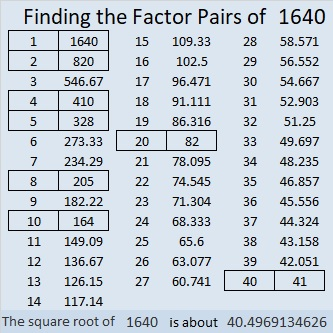# 1640 A Level 2 Flower

### Today’s Puzzle:

Write the numbers from 1 to 12 in both the first column and the top row so that those numbers and the given clues function like a multiplication table.### Factors of 1640:

• 1640 is a composite number.
• Prime factorization: 1640 = 2 × 2 × 2 × 5 × 41, which can be written 1640 = 2³ × 5 × 41.
• 1640 has at least one exponent greater than 1 in its prime factorization so √1640 can be simplified. Taking the factor pair from the factor pair table below with the largest square number factor, we get √1640 = (√4)(√410) = 2√410.
• The exponents in the prime factorization are 3,1 and 1. Adding one to each exponent and multiplying we get (3 + 1)(1 + 1) (1 + 1) = 4 × 2 × 2 = 16. Therefore 1640 has exactly 16 factors.
• The factors of 1640 are outlined with their factor pair partners in the graphic below.### More about the Number 1640:

Since 1640 = 40 x 41, we can be sure that 1640 is the sum of the first 40 even numbers.

1640 is the sum of two squares in two different ways:
38² + 14² = 1640, and
34² + 22² = 1640.

1640 is the hypotenuse of a Pythagorean triple in FOUR different ways:
360-1600-1640, which is 40 times (9-40-41),
672-1496-1640, calculated from 34² – 22², 2(34)(22), 34² + 22²,
but it is also 8 times (84-187-205),
984-1312-1640, which is (3-4-5) times 328, and
1064-1248-1640, calculated from 2(38)(14), 38² – 14², 38² + 14²,
but it is also 8 times (133-156-205).

1640₁₀ = 2222₉ because 2(9³ + 9² + 9¹ + 9⁰) = 2(729 + 81 + 9 + 1) = 2(820) = 1640.
1640₁₀ = 2020202₃ because 2(3⁶ + 3⁴ + 3² + 3⁰) = 2(729 + 81 + 9 + 1) = 2(820) = 1640.